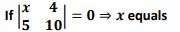# FSC Mathematics MCQs Chapter 2

FSC Math MCQs entry test 2023 NUST NET contains most of the Mathematics MCQs. FSC Part 1 Math book MCQs. HSSC Mathematics questions MCQs. Chapterwise mathematics MCQs for first year 11 class MCQs.

415
Created on By Ali Durrani

Ch 2 Sets, Functions and Groups

1 / 37

Which of the following is true:

2 / 37

Every function is:

3 / 37

If 𝑨 and 𝑩 are disjoint sets , then 𝑨 ∩ 𝑩 =

4 / 37

A diagram which represents a set is called______

5 / 37

The graph of linear function is :

6 / 37

In a proposition if 𝒑 → 𝒒 𝒕𝒉𝒆𝒏 𝒒 → 𝒑 is called

7 / 37

𝑳 ∪ 𝑴 = 𝑳 ∩ 𝑴 then 𝑳 is equal to

8 / 37

Squaring a number is a:

9 / 37

The set of odd numbers between 1 and 9 are

10 / 37

Inverse of any element of a group is:

11 / 37

Every recurring non terminating decimal represents

12 / 37

A set is a collection of objects which are

13 / 37

If 𝒙 ∈ 𝑩′ = 𝑼 − 𝑩 then

14 / 37

The number of all subsets of a set having three elements is:

15 / 37

𝒑 → 𝒒 is called converse of

16 / 37

Total number of subsets that can be formed from the set {𝒙, 𝒚, 𝒛} is

17 / 37

If 𝒙 ∈ 𝑳 ∪ 𝑴 then

18 / 37

𝑹 − {𝟎} is a group 𝒘. 𝒓. 𝒕 the binary operation

19 / 37

Identity element in (𝑪, . ) is:

20 / 37

If 𝑨 ⊆ 𝑩 then 𝒏(𝑨 ∩ 𝑩) =

21 / 37

A compound proposition which is always wrong is called:

22 / 37

The graph of linear function is :

23 / 37

The set of odd numbers between 1 and 9 are

24 / 37

A compound proposition which is always neither true nor false is called:

25 / 37

A compound proposition which is always true is called:

26 / 37

The number of all subsets of a set having three elements is:

27 / 37

Squaring a number is a:

28 / 37

A diagram which represents a set is called______

29 / 37

A set is a collection of objects which are

30 / 37

Inverse of a line is :

31 / 37

Every function is:

32 / 37

The symbol which is used to denote negation of a proposition is

33 / 37

Inverse of any element of a group is:

34 / 3735 / 37

An onto function is also called:

36 / 37

. Truth set of a tautology is

37 / 37

Which of the following is not a binary operation :